# Algebra II : Functions as Graphs

## Example Questions

### Example Question #11 : Introduction To Functions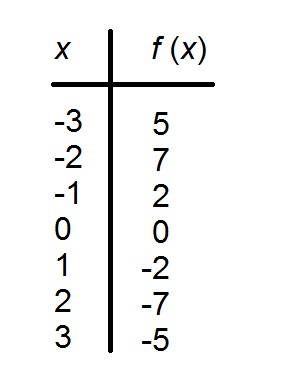The above table refers to a functionwith domain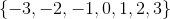.

Is this function even, odd, or neither?

Odd

Even

Neither

Odd

Explanation:

A function is odd if and only if, for everyin its domain,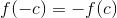; it is even if and only if, for everyin its domain,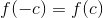. We can see that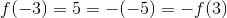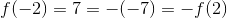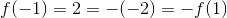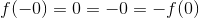It follows thatis an odd function.

### Example Question #12 : Functions And Graphs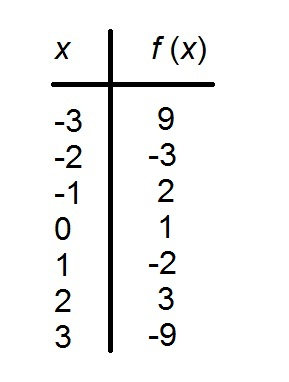The above table refers to a functionwith domain.

Is this function even, odd, or neither?

Even

Neither

Odd

Neither

Explanation:

A function is odd if and only if, for everyin its domain,; it is even if and only if, for everyin its domain,. We can see that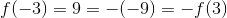;

the function cannot be even. This does allow for the function to be odd. However, ifis odd, then, by definition,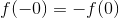, or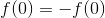and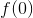is equal to its own opposite - the only such number is 0, so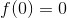.

This is not the case -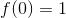- so the function is not odd either.

### Example Question #13 : Functions And Graphs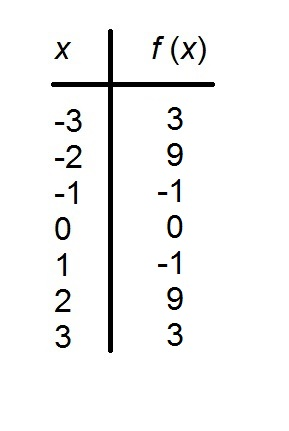The above table refers to a functionwith domain.

Is this function even, odd, or neither?

Neither

Even

Odd

Even

Explanation:

A functionis odd if and only if, for everyin its domain,; it is even if and only if, for everyin its domain,. We can see that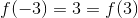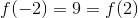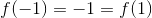Of course,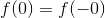.

Therefore,is even by definition.

### Example Question #14 : Functions And Graphs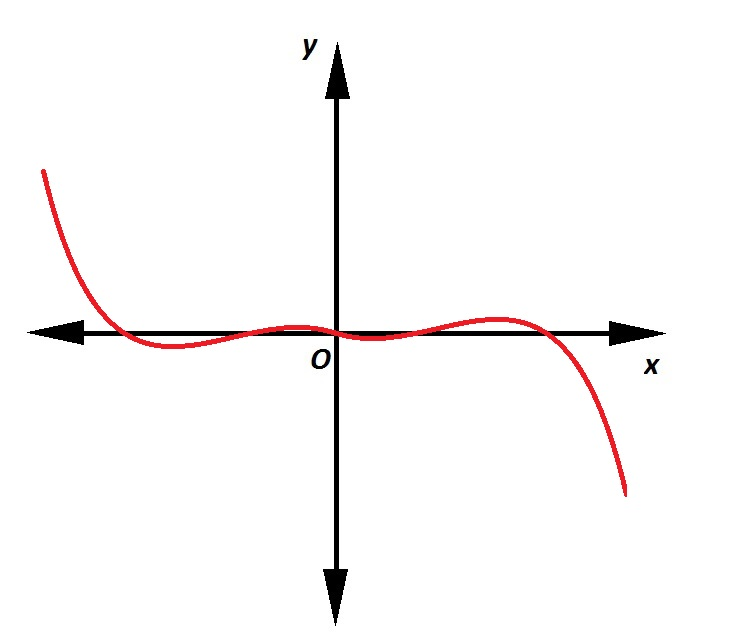Which of the following is true of the relation graphed above?

It is an even function

It is not a function

It is a function, but it is neither even nor odd.

It is an odd function

It is an odd function

Explanation:

The relation graphed is a function, as it passes the vertical line test - no vertical line can pass through it more than once, as is demonstrated below: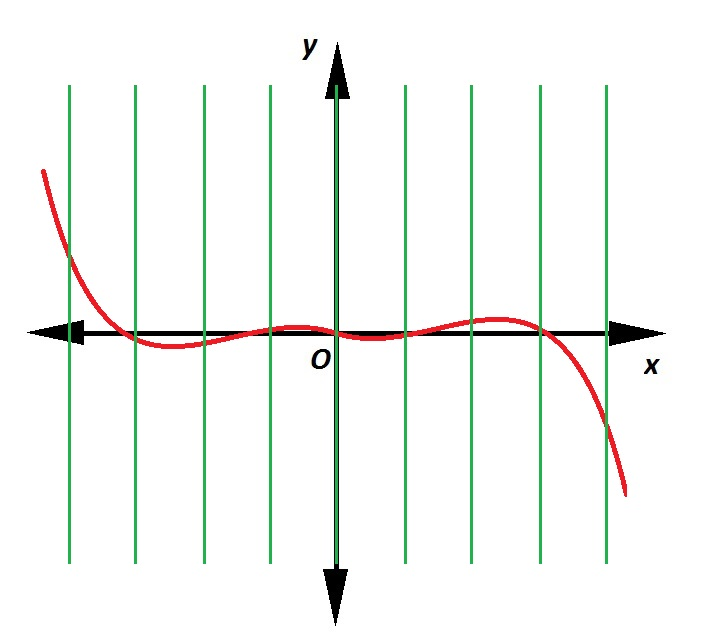Also, it can be seen to be symmetrical about the origin. Consequently, for eachin the domain,- the function is odd.

### Example Question #15 : Functions And Graphs

Which is a vertical asymptote of the graph of the function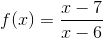?

(a)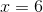(b)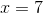(a) only

Both (a) and (b)

(b) only

Neither (a) nor (b)

(a) only

Explanation:

The vertical asymptote(s) of the graph of a rational function such ascan be found by evaluating the zeroes of the denominator after the rational expression is reduced. The expression is in simplest form, so set the denominator equal to 0 and solve for: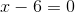The graph ofhas the line of the equationas its only vertical asymptote.

### Example Question #16 : Functions And Graphs

Which of the following is a vertical asymptote of the graph of the function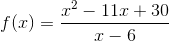?

(a)(b)(a) only

Both (a) and (b)

Neither (a) nor (b)

(b) only

Neither (a) nor (b)

Explanation:

The vertical asymptote(s) of the graph of a rational function such ascan be found by evaluating the zeroes of the denominator after the rational expression is reduced

First, factor the numerator. It is a quadratic trinomial with lead term, so look to "reverse-FOIL" it as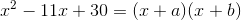by finding two integers with sum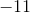and product 30. By trial and error, these integers can be found to beand, so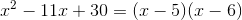Therefore,can be rewritten as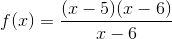.

Cancelling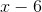, this can be seen to be essentially a polynomial function: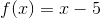,

which does not have a vertical asymptote.

### Example Question #17 : Functions And Graphs

True or false: The graph of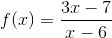has as a horizontal asymptote the graph of the equation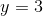.

True

False

True

Explanation:is a rational function whose numerator and denominator have the same degree (1). As such, it has as a horizontal asymptote the line of the equation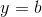, whereis the quotient of the coefficients of the highest-degree terms of its numerator and denominator. Consequently, the horizontal asymptote ofis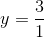,

or.

### Example Question #18 : Functions And Graphs

True or false: The graph of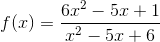has as a horizontal asymptote the graph of the equation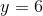.

True

False

True

Explanation:is a rational function whose numerator and denominator have the same degree (2). As such, it has as a horizontal asymptote the line of the equation, whereis the quotient of the coefficients of the highest-degree terms of its numerator and denominator. Consequently, the horizontal asymptote ofis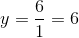or.

### Example Question #19 : Functions And Graphs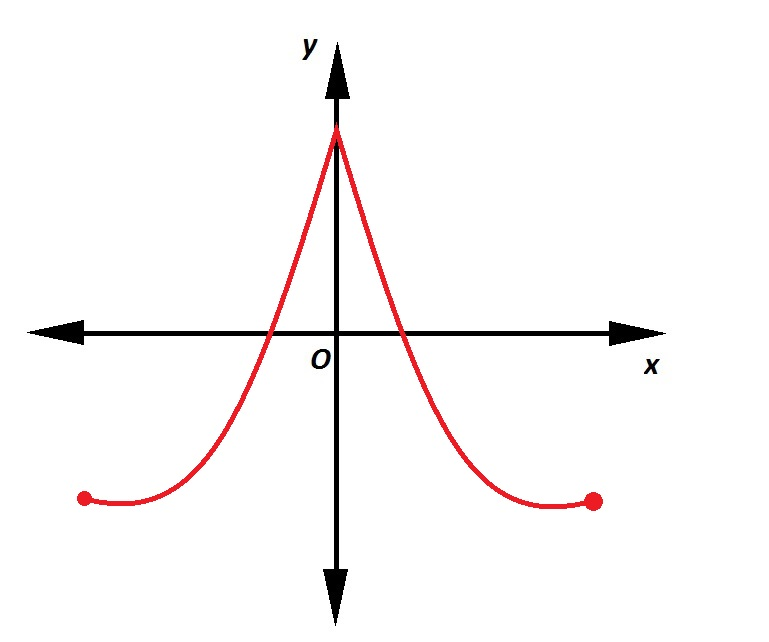Which of the following is true of the relation graphed above?

It is an odd function

It is not a function

It is a function, but it is neither even nor odd.

It is an even function

It is an even function

Explanation:

The relation graphed is a function, as it passes the vertical line test - no vertical line can pass through it more than once, as seen below: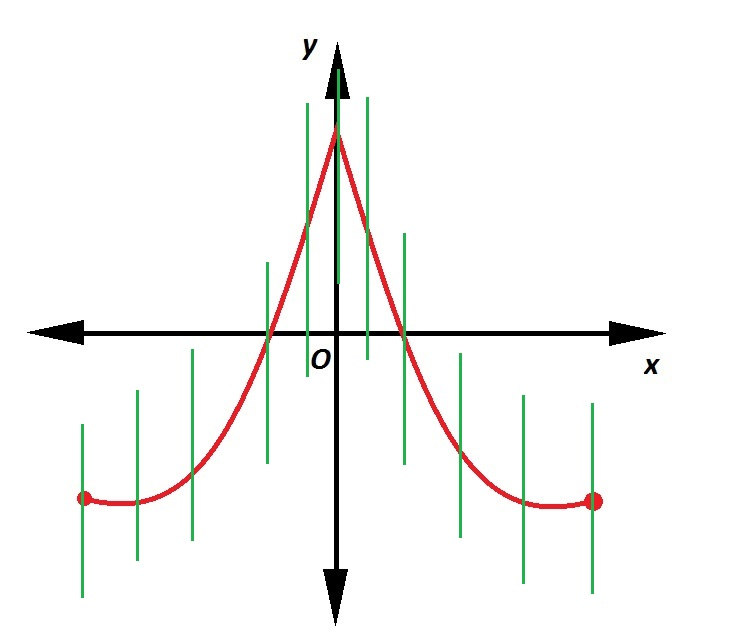Also, it is seen to be symmetrical about the-axis. This proves the function even.

### Example Question #20 : Functions And Graphs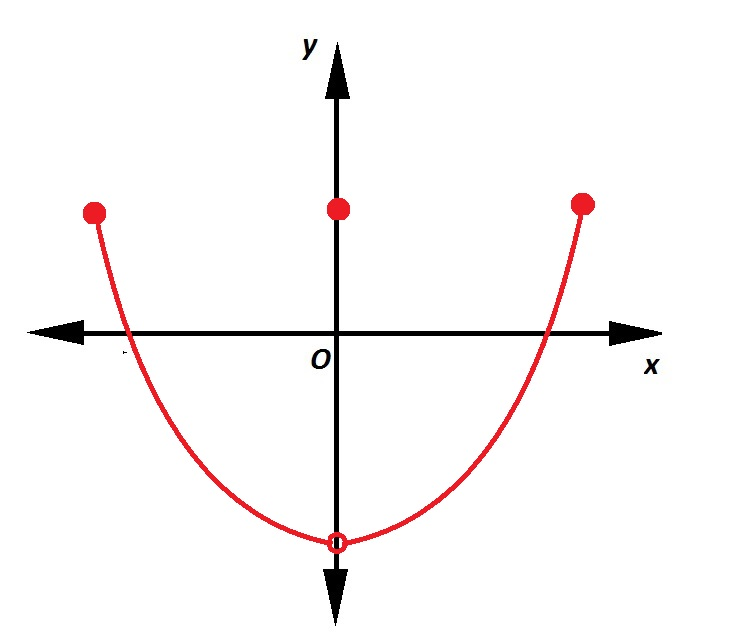Which of the following is true of the relation graphed above?

It is not a function

It is an even function

It is a function, but it is neither even nor odd.

It is an odd function

It is an even function

Explanation:

The relation graphed is a function, as it passes the vertical line test - no vertical line can pass through it more than once, as is demonstrated below: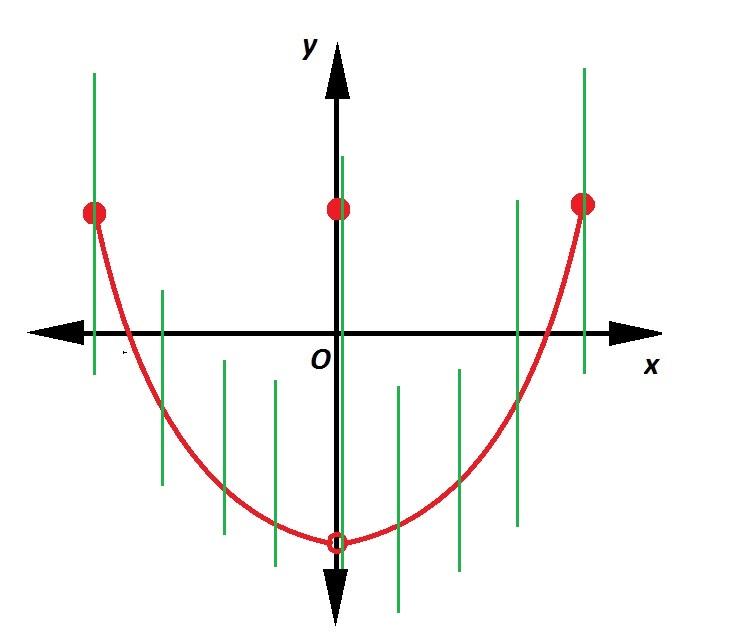Also, it is seen to be symmetrical about the-axis. Consequently, for eachin the domain,- the function is even.

### All Algebra II Resources